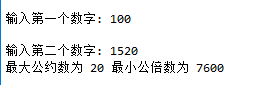2021-05-04 17:35

# python 本题要求两个给定正整数的最大公约数和最小公倍数

7-11 本题要求两个给定正整数的最大公约数和最小公倍数。 (15 分)

``````100
1520``````

``20 7600``
• 写回答
• 关注问题
• 收藏
• 邀请回答

#### 1条回答默认 最新

•已采纳
``````"""该函数返回两个数的最大公约数"""
def hcf(x, y):
# 获取最小值
if x > y:
smaller = y
else:
smaller = x
for i in range(1,smaller + 1):
if((x % i == 0) and (y % i == 0)):
hcf = i
return hcf
"""该函数返回两个数的最小公倍数"""
def lcm(x, y):
#  获取最大的数
if x > y:
greater = x
else:
greater = y

while(True):
if((greater % x == 0) and (greater % y == 0)):
lcm = greater
break
greater += 1

return lcm

# 用户输入两个数字
num1 = int(input("输入第一个数字: "))
num2 = int(input("输入第二个数字: "))

print("最大公约数为",hcf(num1, num2),"最小公倍数为",lcm(num1,num2))``````

运行结果：已采纳该答案
2 打赏 评论